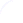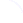Search
 Submission Procedure share: |available in:   PDF (207 kB) PS (222 kB)

get:
 Similar Docs BibTeX Write a comment
get:

DOI:   10.3217/jucs-016-01-0140

### An Approach to Generation of Decision Rules

Zhang Mingyi (Southwest University, China)

Li Danning (Guizhou Academy of Sciences, China)

Zhang Ying (Guizhou Academy of Sciences, China)

Abstract: Classical classification and clustering based on equivalence relations are very important tools in decision-making. An equivalence relation is usually determined by properties of objects in a given domain. When making decision, anything that can be spoken about in the subject position of a natural sentence is an object, properties of which are fundamental elements of the knowledge of the given domain. This gives the possibility of representing the concept related to a given domain. In general, the information about a set of the objects is uncertain or incomplete. Various approaches representing uncertainty of a concept were proposed. In particular, Zadeh?s fuzzy set theory and Pawlak?s rough set theory have been most influential on this research field. Zadeh characterizes uncertainty of a concept by introducing a membership function and a similarity (fuzzy equivalence) relation of a set of objects. Pawlak then characterizes uncertainty of a concept by union of some equivalence classes of an equivalence relation. As one of particular important and widely used binary relations, equivalence relation plays a fundamental role in classification, clustering, pattern recognition, polling, automata, learning, control inference and natural language understanding, etc.  An equivalence relation is a binary relation with reflexivity, symmetry and transitivity. However, in many real situations, it is not sufficient to consider equivalence relations only. In fact, a lot of relations determined by the attributes of objects do not satisfy transitivity. In particular, information obtained from a domain of objects is not transitive, when we make decision based on properties of objects. Moreover, the information about symmetry of a relation is mostly uncertain. So, it is needed to approximately make decision and reasoning by indistinct concepts. This provokes us to explore a new class of relations, so-called class of fuzzy semi-equivalence relations. In this paper we introduce the notion of fuzzy semi-equivalence relations and study its properties. In particular, a constructive method of fuzzy semi-equivalence classes is presented. Applying it we present approaches to the fuzzyfication of indistinct concepts approximated by fuzzy relative and semi-equivalence classes, respectively. And an application of the fuzzy semi-equivalence relation theory to generate decision rules is outlined.

Keywords: approximate definability of indistinct concepts, fuzzy semi-equivalence relation, fuzzy theory, rough set

Categories: H.3.1, H.3.2, H.3.3, H.3.7, H.5.1